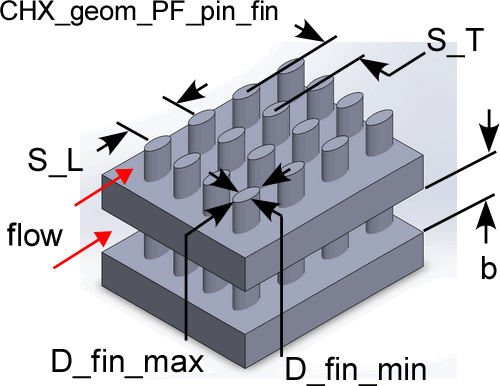Contents

PF pin-fin geometryThe procedure:

CHX_Geom_PF_pin_fin(TypeHX\$: S_T, S_L, D_fin_min, D_fin_max, b, D_h, theta, beta, A_fin\A)

Provides the geometric data associated with a plate finned - pin-fin type compact heat exchanger surface.  These data are from Kays and London (1994).

Inputs:

The only input is the string identifying the geometry TypeHX\$

AP-1: 'PF_pin-fin_AP1'

AP-2: 'PF_pin-fin_AP2'

PF-3: 'PF_pin-fin_PF3'

PF-4(F): 'PF_pin-fin_PF4F'

PF-9(F): 'PF_pin-fin_PF9F'

PF-10(F): 'PF_pin-fin_PF10F'

Outputs:

S_T = transverse fin spacing (m or ft)

S_L = longitudinal fin spacing (m or ft)

D_fin_min = minimum fin diameter (m or ft)

D_fin_max = maximum fin diameter (m or ft), same as D_fin_min for round fins

b = plate spacing (fin length) (m or ft)

D_h = hydraulic diameter defined as 4 x minimum flow area x length/heat transfer area (m or ft)

theta = minimum flow area/frontal area for finned surface side only

beta = heat transfer area/volume (m^2/m^3 or ft^2/ft^3) for finned surface side only

A_fin\A = heat transfer area on fins/heat transfer area for finned surface side only

Example

\$UnitSystem SI Mass J K Pa

TypeHX\$='PF_pin-fin_AP-1'

Call chx_geom_pf_pin_fin(TypeHX\$: S_T, S_L, D_fin_min, D_fin_max, b, D_h, theta, beta, A_fin\A)

{Solution is:

S_T = 0.003175 [m]

S_L = 0.003175 [m]

D_fin_min = 0.00102 [m]

D_fin_max = 0.00102 [m]

b = 0.0061 [m]

D_h = 0.004404 [m]

theta = 0.6791

beta = 616.8 [m^2/m^3]

A_fin\A = 0.512}

Related procedures include:

Nondimensional Functions

Coefficient of Heat Transfer

Pressure Drop

Compact Heat Exchangers